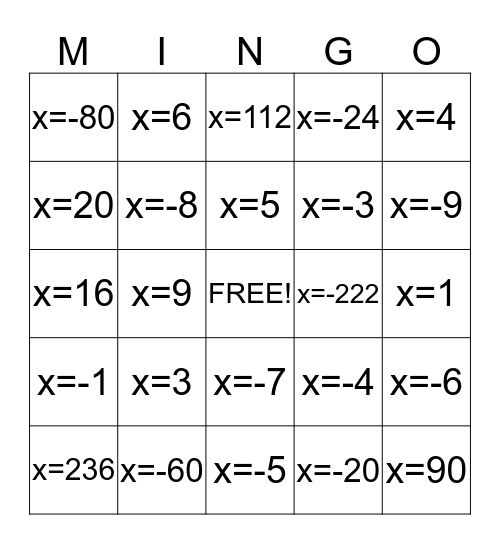# Two Step EquationsThis bingo card has a free space and 24 words: x=3, x=-60, x=5, x=236, x=4, x=-80, x=20, x=6, x=-222, x=-7, x=-3, x=16, x=-4, x=-6, x=-8, x=-20, x=1, x=112, x=-9, x=9, x=90, x=-1, x=-24 and x=-5.

## Play Online